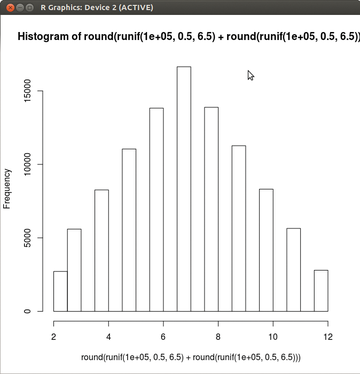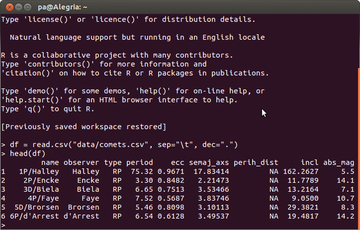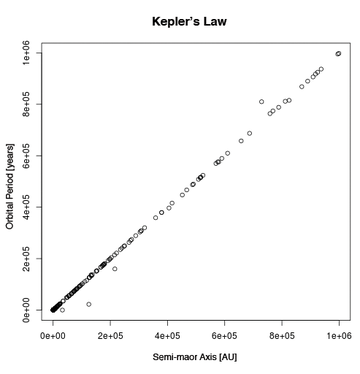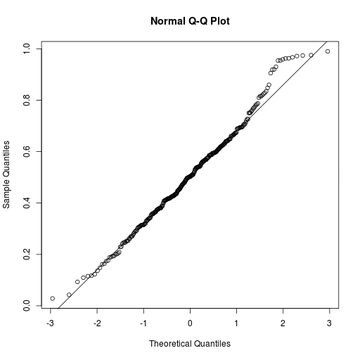# On Track

#### Results Oriented

R is a free implementation of the statistics programming languages S and S-Plus. Like the S languages, R facilitates the process of performing statistical computations and generating matching graphics (see the "Interactive R" box for more information). R is licensed under the GPL, and the current version is 3.1.2. Listing 4 shows the installation of the current version on Ubuntu 12.04.

Interactive R

Like Python, R can also be launched in an interactive shell using the `R` command. You can then experiment in the shell. The expression

`hist(round(runif(100000, 0.5, 6.5)) + round(runif(100000, 0.5, 6.5)))`

describes an experiment with two dice. The `runif()` function determines two vectors with 100,000 equally distributed random numbers from the interval 0.5 to 65 each, which `round()` then rounds to 1 and 6.

Each vector component simulates a throw of the dice. The call to `hist()` creates a histogram like the one in Figure 3 for the sum of the vectors. The sum of the dots is on the x -axis and the frequency of occurrence is on the y -axis. As you might expect, the result is a symmetrical distribution about the value of `7`.

The sample data for the comets can be read and evaluated interactively, even though the results are not too impressive as a graph (Figure 4). The command Programmers will be familiar with this feature from Python, where it is used to test functions.

`df = read.csv("data/comets.csv", sep="\t", dec=".")`

uses the `csv()` method to load the data into the R session from the `data/comtes.csv` file; it converts the data to a dataframe type object and stores the object in the variable `df`. The named attribute, `sep="\t"`, from the call to the method, separates the columns on the tab character; `dec="."` defines a period as the decimal separator. R takes the attribute names from the first line in the CSV file. The expression `head(df)` finally lists the header of `df` as a table (Figure 4).Figure 3: Simulation of a dice experiment in R.Figure 4: An interactive R session lists the sample data as a table.

Listing 4

Installing R on Ubuntu 12.04

```sudo apt-key adv --keyserver keyserver.ubuntu.com --recv-keys E084DAB9
sudo apt-get update
sudo apt-get install r-base r-base-dev```

Like JavaScript, R exclusively stores values in objects, which it binds lexically to an environment or a closure object. The most commonly used data types are vectors, matrices, lists, and data frames. R processes the values with the help of various built-in function and operators, typical control structures, and user-defined functions and operators. The Comprehensive R Archive Network (Cran ) also offers many modules for R.

#### R Interface

The sample application uses the Python Rpy2  package as an interface to R. Rpy2 passes in the data and function calls via R's C interface to an embedded R process and accepts the results. Rpy2 also provides an object-based interface to R in the form of the `robjects` module; it includes a separate Python class for each R object type.

The R functions work with the objects of the Python classes as they do with native R objects: For example, they convert the Python expression `robjects.r. length(robjects.FloatVector([1.0, 2.0])` to the expression `length(c(1.0, 2.0))` for the R process and evaluate it in the process. Rpy2 is licensed under the GPLv2 and is currently at version 2.5.4. Listing 5 installs the current version of Rpy2 on Ubuntu 12.04.

Listing 5

Installing Rpy2

```sudo apt-get install python-dev
sudo easy_install rpy2```

#### Reports

The Python reports in the sample application all use the `reporter` class from Listing 6 (lines 5 to 21) to read data from the MongoDB database and convert it to a Python dataframe object with the help of R. Their constructor adds the name of a MongoDB database and a data collection, a list of attributes, and an optional selector in its list of parameters (line 6).

Listing 6

www/rreporter.py

```01 from pymongo import MongoClient
02 import rpy2.robjects as ro
03 import json
04
05 class reporter:
06   def __init__(self, name, collec, keys, fexpr=None):
07     count = 0
08     doc = None
09     for doc in MongoClient()[name][collec].find(fexpr):
10       try:
11         if count == 0:
12           ro.r('df = %s' %ro.Dataframe({key: float(doc[key]) for key in keys}).r_repr())
13           count += 1
14         else:
15           ro.r('df[nrow(df)+1,] = %s' %ro.FloatVector([float(doc[key]) for key in keys]).r_repr())
16       except:
17         pass
18     self.df = ro.r('df')
19
20   def respond(self, obj):
21     print "Content-Type: application/json;charset=utf-8\n\r\n\r%s" %json.dumps(obj)```

Lines 9 through 18 iterate against all entries of a database cursor, which the call to the `find()` method in line 9, generates from the queried data collection.

During the first loop, the `Dataframe` constructor (line 12) generates an object from the dictionary passed into it. The generator expression creates the dictionary in the curly brackets from the list of attributes, the `keys` call parameter, and the `keys` attribute values from the current cursor entry from the `doc` variable. After instantiation, the `r_repr()` method converts the object into a string and replaces `%s` in `df = %s` with it.

The embedded R process evaluates the object as a dataframe object with the help of the `r()` method, finally saving it in the `df` variable. In a similar way, the second R side loop iteration in line 15 adds `df` to the cursor entries it reads. This time, the `r()` method dumps the values into a `FloatVector` type object and substitutes them into the R expression `df[nrow(df)+1,] = %s`.

Line 18 saves the dataframe created in this way as a Python object in the `self.df` component. The Python scripts use `respond()` as of line 20 to transfer the resulting URL to the web server like a CGI response. Line 21 appends the Python object to the header in JSON format.

Listing 7 generates the first report. The report shows the relative frequency of the ellipse, parabola, and hyperbola orbit types as a pie diagram (Figure 1). In the shebang (`#!`) line, the script first selects Python as the executing instance. The script uses the `importr()` method to retrofit R modules and then imports the `reporter` class from Listing 6 and the `robjects` module.

Listing 7

www/bahntypen.py

```01 #!/usr/bin/env python
02 from rpy2.robjects.packages import importr
03 from rreport import reporter
04 import rpy2.robjects as ro
05
06 devs = importr('grDevices')
07
08 main = "Distribution of Orbit Types"
09 path = "tmp/bahntypen.png"
10
11 rep = reporter("galaxy", "comets", ["ecc"])
12 num = ro.r.nrow(rep.df)
13 vals = ro.r.table(ro.r.cut(rep.df.rx2(1), [0, 0.9999, 1.0001, 2]))
14
15 label = lambda label, i: "%s %s %%" %(label, round((vals[i]*100)/num))
16 labels = [label("Ellipse", 0), label("Parabola", 1), label("Hyperbola", 2)]
17 col = ro.r.rainbow(len(labels))
18
19 devs.png(file=path, width=512, height=512)
20 ro.r.pie(vals, labels=labels, col=col, main=main)
21 devs.dev_off()
22
23 rep.respond({"fileref":path})```

Line 6 uses `importr()` to integrate the export mechanism for graphs into the running R process. The `main` variable in line 8 stores the report title; the Python script uses `path` in line 9 to create the path for the graph to be created relative to its own storage location. Line 11 generates an object of the `reporter` class as an instance and stores it in the `rep` variable. The call to the constructor uses the MongoDB name (`galaxy`) and the data collection name (`comets`). The list in the third call parameter requests the eccentricity values.

Line 12 uses `nrow` to count the records in the dataframe object, `rep.df`, and stores the results in the `num` variable. The `rx2()` first copies the values from the first column of `rep.df` to a `FloatVector` type object. The `cut()` maps the vector components to one of the three R factors, `[0-0.9999)`, `[0.999-1.0001)`, or `[1.0001-2)` (these are semi-open intervals), and uses `table()` to create a contingency table from the resulting vector. The `vals` variable stores the table.

The lambda function in line 15 formats the pie labels using a designator in the `label` variable and an index in `i`. The function references `i`, `vals`, and `num` compute the relative frequency of the orbit type as a percentage.

By repeatedly calling the lambda function, line 16 generates and stores labels in the `labels` field; line 17 generates a color index for the pie segments in three colors. Line 19 uses `png()` to open the file from the `path` variable to store the figures. Line 20 uses `pie()` to create the pie chart.

The function imports the `vals` contingency table with the graphics options from lines 16, 17, and 18. Line 21 stops the output to the file before `rep.respond()` has sent the relative URL for the graph to the browser as an HTTP response.

The `Kepler3.py` report in Listing 8 verifies the validity of Kepler's third law , according to which the square of the orbit time T of a celestial body moving in an elliptical orbit about the sun is proportional to the cube of its semi-major axis: T^2 ~ a^3. If you plot a^3 vs. T^2, the result should be a straight line according to Kepler.

Listing 8 differs from Listing 7 as of line 11 in that it loads the values of the semi-major axis and the orbit time into the dataframe object. The last parameter in the constructor call in line 11 contains the MongoDB selector `{"semaj_axs":{"\$lt":100}}`, which the `reporter` class from Listing 6 passes to the `find()` method. The selector only selects data from comets with a large half-axis of less than 100AU.

Listing 8

www/Kepler3.py

```01 #!/usr/bin/env python
02 from rpy2.robjects.packages import importr
03 from rreport import reporter
04 import rpy2.robjects as ro
05
06 devs = importr('grDevices')
07
08 main = "3. Kepler's Law"
09 path = "tmp/Kepler3.png"
10
11 rep = reporter("galaxy", "comets", ["semaj_axs", "period"], {"semaj_axs":{"\$lt":100}})
12 x_lab = "Semi-major axis / [AU]"
13 x_vals = ro.FloatVector([x**3 for x in rep.df.rx2(1)])
14
15 y_lab = "Orbital Period / [Years]"
16 y_vals = ro.FloatVector([x**2 for x in rep.df.rx2(2)])
17
18 devs.png(file=path, width=512, height=512)
19 ro.r.plot(x_vals, y_vals, xlab=x_lab, ylab=y_lab, main=main)
20 devs.dev_off()
21
22 rep.respond({"fileref":path})```

In line 12, the variable `x_lab` stores the label for the x -axis. Line 13 accepts the generator expression with the values for the cube of the semi-major axis and passes the results on to the constructor of the `FloatVector` class, which stores them in the `x_vals` variable.

Lines 15 and 16 repeat the procedure for the orbit time and the y -axis. The `plot()` function creates a dot diagram, which plots `x_vals` vs. `y_vals` (Figure 5). The dots are in a straight line, as expected.Figure 5: The comets confirm Kepler's third law.

Listing 9 compares the distribution of the eccentricities for regular periodic comets (`{"type":"RP}`) with the normal distribution using a quantile-quantile plot `qqnorm()` (line 14). The call to `qqline()` retroactively draws a model straight line in the graph. The closer the quantile of the measure distribution matches that of the normal distribution, the closer the points are to the model straight line that halves the angle (Figure 6).

Listing 9

www/eccdistr.py

```01 #!/usr/bin/env python
02
03 from rreport import reporter
04 from rpy2.robjects.packages import importr
05 import rpy2.robjects as ro
06
07 devs = importr('grDevices')
08
09 path = "tmp/qqnorm.png"
10
11 rep = reporter("galaxy", "comets", ["ecc"], {"type":"RP"})
12
13 devs.png(file=path, width=512, height=512)
14 ro.r.qqnorm(rep.df.rx2(1))
15 ro.r.qqline(rep.df.rx2(1))
16 devs.dev_off()
17
18 rep.respond({"fileref":path})```Figure 6: Not a normally distributed eccentricity.

The distribution of the eccentricities is similar to the normal distribution for the most part but deviates too substantially from it for larger values. These comets might be subject to major scatter processes.

Express-Checkout as PDF
Price \$2.95
(incl. VAT)

SINGLE ISSUES

SUBSCRIPTIONS